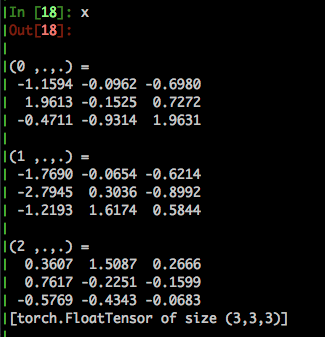# Keep the max value of the array and 0 the others

Hello and good evening,

I’ve searched a lot but with no significant result so far so I’m posting in this forumI have a Tensor in the form of :
(0 ,.,.) =
1.0858 -3.2292 -0.6477

(1 ,.,.) =
3.3863 -2.3600 -2.6776

(2 ,.,.) =
-2.3978 -2.0387 2.0728

and i want to transform it in a way that all the max values of each row will get the numer 1 and the others the number 0.
Any help would be very apprieciatedTry the following:

``````x == x.max(dim=0)
``````

well not exactly… you see the correct output would be something

(0 ,.,.) =
1 0 0

(1 ,.,.) =
1 0 0

(2 ,.,.) =
0 0 1

with this i only get the max results and i can’t keep the shape of the array i need. ( I don’t want to be forced to do this with a for loop)

Could you provide a solid example of a tensor? ie how many dimensions does this tensor have?

It has 3 dimensions it’s a 30x1x3 tensor

Okay, so you have something like this:What should the output be?

(0 ,.,.) =

0 0 1
1 0 0
0 0 1

(1 ,.,.) =

0 0 1
0 1 0
0 1 0

(2 ,.,.) =
0 1 0
1 0 0
0 0 1

Thank you. Try the following:

``````view = x.view(-1, 3)
(view == view.max(dim=1, keepdim=True)).view_as(x)
``````
3 Likes

Just saved me!!! thanks a lotif temp is your tensor this line do what you want